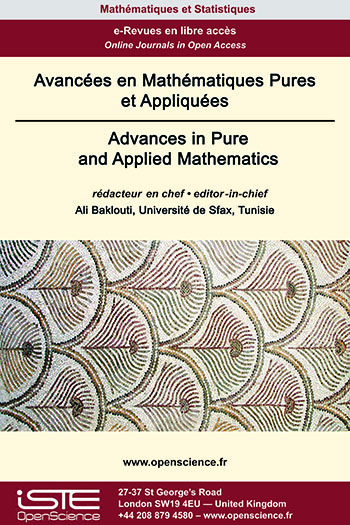# Vol 14 - Issue 1 (January 2023)

## List of Articles

Constrained Hitting Set and Steiner Tree in SCk and 2K2-free Graphs

Strictly Chordality-k graphs (SCk) are graphs which are either cycle-free or every induced cycle is of length exactly ${k, k \geq 3}$. Strictly chordality-3 and strictly chordality-4 graphs are well known chordal and chordal bipartite graphs, respectively. For ${k \geq 5}$, the study has been recently initiated in  and various structural and algorithmic results are reported. In this paper, we study SCk graphs in the algorithmic front and the study concerns the class of graphs where ${k \geq 5}$. We show that recognizing vertex cycle ordering (VCO) for SCk, ${k \geq 5}$ graphs, maximum independent set (MIS), minimum vertex cover, minimum dominating set, feedback vertex set (FVS), odd cycle transversal (OCT), even cycle transversal (ECT) and Steiner tree problem are linear time solvable on SCk graphs, ${k \geq 5}$. We next consider 2K2-free graphs and discussed the algorithmic problems such as FVS, OCT, ECT and Steiner tree problem on the subclasses of 2K2-free graphs.

Zak transform for groupoids with abelian isotropy

Let 𝒢 be a second countable locally compact Hausdorff groupoid with abelian isotropy groups and 𝓛 be a lattice bundle in the isotropy subgroupoid 𝒢 ’ of 𝒢. In this paper, we define a Zak transform on 𝒢 relatively to 𝓛 and study some of its properties. Moreover, we use Zak transform to obtain some characterizations of the cyclic subbundle of the left regular representation of 𝒢 restricted to 𝓛.

Multiplicative Jordan type higher derivations of unital rings with non trivial idempotents

Suppose R is a non-zero unital associative ring with a nontrivial idempotent "e". In this paper, we prove that under some mild conditions every multiplicative jordan n-higher derivations on R is additive. Moreover, at the end of the paper, we have presented some applications of multiplicative Jordan n-higher derivations on triangular rings, nest algebra, upper triangular block matrix algebra, prime rings, von Neumann algebras.

An inverse problem for the Schrödinger equation with Neumann boundary condition

This article concerns the inverse problem of the recovery of unknown potential coefficient for the Schrödinger equation, in a bounded domain of ℝn with non-homogeneous Neumann boundary condition from a time-dependent Dirichlet boundary measurement. We prove uniqueness and Lipschitz stability for this inverse problem under certain convexity hypothesis on the geometry of the spatial domain and under weak regularity requirements on the data. The proof is based on a Carleman estimate in  for Schrödinger equations and its resulting implication, a continuous observability inequality.### Other issues :

2020

Volume 20- 11

Issue 1 (May 2020)
Issue 2 (September 2020)

2021

Volume 21- 12

Issue 1 (January 2021)
Issue 2 (May 2021)
Issue 3 (Special AUS-ICMS 2020)
Issue 4 (September 2021)

2022

Volume 22- 13

Forthcoming papers

Issue 1 (January 2022)
Issue 2 (March 2022)
Issue 3 (June 2022)
Issue 4 (September 2022)

2023

Volume 23- 14

Issue 1 (January 2023)
Issue 2 (Special CSMT 2022)